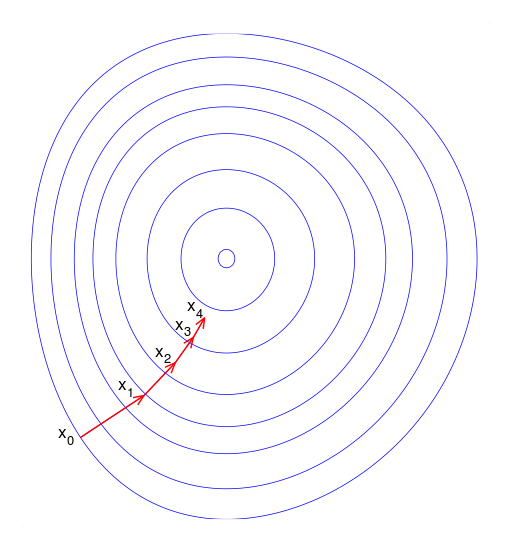Vectorized Implementation

Gradient descent algorithm is one of the most popular optimization algorithms for finding optimal parameters for the model. Goal is to find the parameter which minimize the cost function.

(Full Source Code: https://github.com/SSaishruthi/Gradient_Descent_Algorithm_Using_Numpy)

Process

Local gradient of the error function with respect to the parameter is measured and it goes in the direction of decreasing gradient. Cost function should decrease after every iteration. When the decrease in cost function is less than 10^ (-3), it indicates that minimum point is approaching or very near.

Learning Rate

Learning rate is the one of the important hyper-parameters that controls how big the steps are during gradient descent. Smaller the learning rate, algorithm takes smaller steps in the process of reaching global minimum. If the learning rate is large, it may overshoot minimum and fail to converge. At times, it may even diverge.

Feature Scaling

If there are more than one feature, then feature scaling will help gradient descent to converge quickly. Two feature scaling methods can be applied.

1. Normalization — This is used to adjust the differences among attributes in terms of frequency of occurrence, mean, range and variance. Replace x(i) with x(i) — Mean(x).

2. Standardization — This is done by subtracting the mean and dividing by standard deviation.

Gradient Descent for linear regression

Equation

Y(pred) = b0 + b1*x

Error Function to be minimized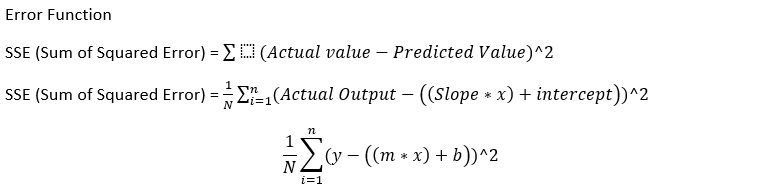Figure 2: Error Function for linear regression

Derivative of error function with respect to the parameters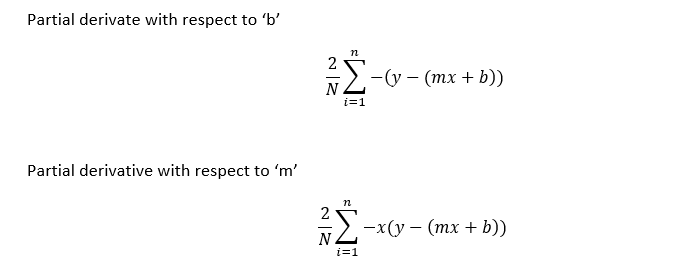Figure 3: Derivative of cost function with respect to the parameter

Three types of gradient descent algorithms are discussed below

1. Batch Gradient Descent
2. Stochastic Gradient Descent
3. Mini-batch Gradient Descent

Flow of the algorithm

As we approach minimum, gradient descent will take only small steps. There is no need to decrease learning rate over time.

def model_optimize(w,b,X,Y):
#
m = X.shape
#
final_result = np.dot(w, X.T) + b
cost = (1/m)*np.sum((Y.T - final_result) ** 2)
#
dw = (-2/m)*np.sum((np.dot(X.T,(Y.T - final_result).T)))
db = (-2/m)*np.sum(((Y.T - final_result)))
#
grads = {"dw": dw, "db": db}

def gradientUpdate(w,b,X, Y, learning_rate, no_iterations):
costs = []
for i in range(no_iterations):
#
grads, cost = model_optimize(w,b, X,Y)
#
#Weight Update
w = w - (learning_rate*dw)
b = b - (learning_rate*db)
#

costs.append(cost)
#

coeff = {"w": w, "b": b}
gradient = {"dw": dw, "db": db}

return coeff, gradient, costs

Cost function with respect to number of iteration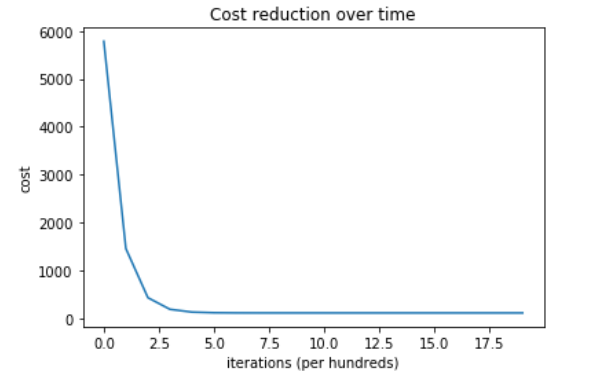Figure 5: Batch gradient descent cost reduction with respect to number of iterations

Flow

Cost function go up and down. There is only gradual decrease in the cost function on an average.

This can help in coming out of local minimum and chances of finding global minimum is not as perfect as batch gradient method. But by decreasing learning rate over the time can help reaching the perfect minimum point.

Process of changing the learning rate at every step is called as simulated annealing. The function which determines the learning rate is called as learning schedule.

def stochasticUpdate(w,b,X, Y, learning_rate, no_iterations):
#
n_points = X.shape
#
for i in range(no_iterations):
for i in range(n_points):
index = np.random.randint(n_points)
x_pt = X[index:index+1]
y_pt = Y[index:index+1]
grads, cost = model_optimize(w,b, x_pt,y_pt)
#
#Weight Update
w = w - (learning_rate*dw)
b = b - (learning_rate*db)
#
costs.append(cost)
#

coeff = {"w": w, "b": b}
gradient = {"dw": dw, "db": db}

return coeff, gradient, costs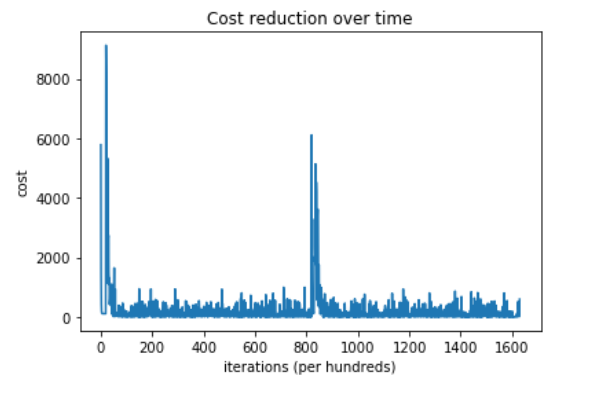Figure 7: Cost function with respect to number of iteration

As discussed, cost function goes up and down for every iteration

Gradient descent is performed on small random sets. It is Less erratic. It can get quite closer to minimum as they reduce variance of parameter update. This leads to more stable updates.

def miniBatchUpdate(w,b,X, Y, learning_rate, no_iterations):
#
n_points = X.shape
#
for i in range(no_iterations):
X, y = shuffle(x_train, y_train)
x_random = X[:40]
y_random = y[:40]

grads, cost = model_optimize(w,b, x_random,y_random)
#
#Weight Update
w = w - (learning_rate*dw)
b = b - (learning_rate*db)
#
costs.append(cost)
#

coeff = {"w": w, "b": b}
gradient = {"dw": dw, "db": db}

return coeff, gradient, costs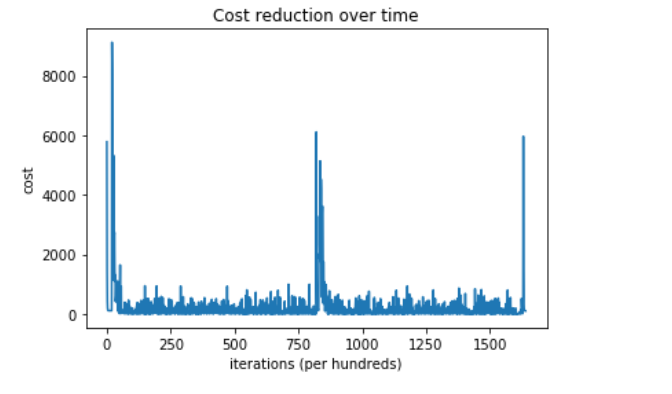Figure 9: Cost Function with respect to number of iterations

Overall performance Comparison

(Full Source code: https://github.com/SSaishruthi/Gradient_Descent_Algorithm_Using_Numpy)Source: Deep Learning on Medium